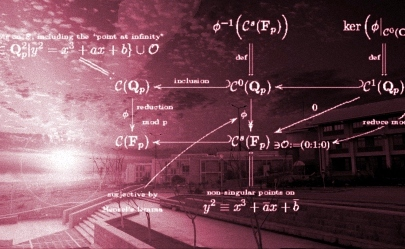Courses

Note: Course webpages are hosted on ODTÜ-Class.

METU NCC Mathematics 2021-22 Fall Term Courses

 MAT 100 Precalculus (ODTÜ-Class) MAT 101 Mathematics for Social Sciences (ODTÜ-Class) MAT 119 Calculus with Analytic Geometry (ODTÜ-Class) MAT 120 Calculus for Functions of Several Variables (ODTÜ-Class) MAT 210 Applied Mathematics for Engineers (ODTÜ-Class) MAT 219 Introduction to Differential Equations (ODTÜ-Class) MAT 260 Basic Linear Algebra (ODTÜ-Class)